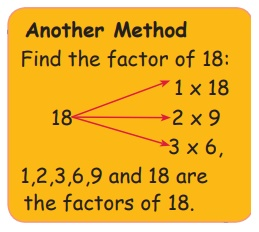Home | | Maths 5th Std | Factors and multiples

# Factors and multiples

Kavitha brought 12 laddus from her home. She gave one laddu for each friend. She was able to give laddus to 12 friends without any remaining.

Factors and multiples

Kavitha brought 12 laddus from her home. She gave one laddu for each friend. She was able to give laddus to 12 friends without any remaining.

If she gives 2 for each, she could give to 6 friends.

If she gives 3 for each, She could give to 4 friends. Like this how can she give laddus so that no laddu would be remaining? She can give each 4, 6, 12.

Numbers that divide 12 without remaining are 1, 2, 3, 4, 6 and 12.

The numbers which can divide a number without leaving a remainder is called its factor.

Try this

Find the Factors:

Factors of 4 are 1, 2 and 4.

Factors of 10 are 1, 2, 5 and 10.

Factors of 16 are 1, 2, 4, 8 and 16.

Factors of 18 are 1, 2, 3, 6, 9 and 18.

Factors of 20 are 1, 2, 4, 5, 10 and 20.

Factors of 24 are 1,2, 3, 4, 6, 8, 12 and 24.

Factors of 42 are 1, 2, 3, 6, 7, 14, 21 and 42.Tags : Numbers | Term 2 Chapter 2 | 5th Maths , 5th Maths : Term 2 Unit 2 : Numbers
Study Material, Lecturing Notes, Assignment, Reference, Wiki description explanation, brief detail
5th Maths : Term 2 Unit 2 : Numbers : Factors and multiples | Numbers | Term 2 Chapter 2 | 5th Maths